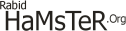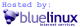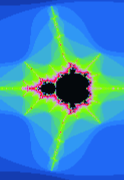Fractal TutorialMain
Home
Guest-Globe
Site Stats

Photos
Kit Car
Index

Geek Stuff
Hardware
Software
3D Scanning
Code Wars
Java
Visuals
R2 Vis
R4 Vis
Tutorials

Feedback
Forum
Contact MeFractals
Fractals are a very interesting phenomena, most are infinitely complex, meaning the more you zoom in, the more detail there is waiting for you. Hopefully, I can explain how you make some fractals here...
Nearly all Fractals are made by a process of iteration, and this is where the link between them and chaos theory comes into play. Basically, you can't write a complete formula for a chaotic system, instead you have to simulate it, doing one step at a time (iteration). Iteration is basically taking the output from a formula, and putting it back into the formula again and again. e.g. If I used Y=2X, and started off with x as 1, I'd get the following series:
```y = 2x = 2
y = 2x = 4
y = 2x = 8
y = 2x = 16
y = 2x = 32
```Probably the easiest kind of fractal to make is the sierpinski gasket. It works on some very simple rules. First, you have 3 points, each one is the corner of an equilateral triangle. You then have a point (I'll call it A) which you can put anywhere in the triangle, but the middle's probably the best place to start. Then you just select a point at random, and move A halfway between A's old position and that of the corner you selected. Then you just leave a dot on the screen, do it a hell of a lot of times, and you get the picture to the left (The colour of the dots were the distance the point had to move each time). The source for the program is below, just type it in in Turbo Pascal:
 ``` uses crt,dos; const GasketSide=3; {Amount of sides (3=triangle) Odd numbers seem to work, even one's just fill up the shape} var x,y,r,c:integer; a:array [1..GasketSide,0..1] of integer; {Stores the X and Y Co-ordinates of the edges of the shape} Begin randomize; {Create a new set of random numbers} Writeln('Creating Lookup...'); for r:=1 to GasketSide do {This fills up the array, it uses Sin and Cos to produce points on a circe} Begin a[r,0]:=160+round(95*sin(r*2*pi/GasketSide)); a[r,1]:=100-round(95*cos(r*2*pi/GasketSide)); End; Writeln('Init Variables...'); x:=160; {Where the point starts off... The middle of the screen} y:=100; asm {Initialise screen in 320x200 mode} mov ax,13h int 10h end; port[\$3c8]:=0; {Set up the video palette} port[\$3c9]:=0; {Color 0 = black} port[\$3c9]:=0; port[\$3c9]:=0; for r:=1 to 255 do {Colors 1-255 blend from blue to red} Begin port[\$3c9]:=r div 4; port[\$3c9]:=0; port[\$3c9]:=63-(r div 4); End; repeat r:=random(GasketSide)+1; {Guess which corner to move to} c:=round(sqrt(sqr(abs(a[r,0]-x))+sqr(abs(a[r,1]-y)))*128 / 95); {The line above figures out a colour using pythagoras, although you could just replace the whole lot by c:=1; if you only wanted a blue fractal} x:=(a[r,0]+x) div 2; {Move half-way between present point and shape corner} y:=(a[r,1]+y) div 2; mem[\$A000:word(x)+(word(y)*320)]:=c; {Put the point onto the screen} until keypressed; {Keep adding to it until a key is pressed} readkey; textmode(lastmode); {Go back into text mode} End. ```

Another Interesting point is that a fractal can be created that looks exactly the same using just pascal's triangle. This is where a triangle is made, where each point is the sum of the two points above. If you make even numbers black, and odd ones white, you get the same triangle. Source is below, just put it in Turbo Pascal and to should run.

 ``` uses crt,dos; var a:array [0..127,0..127] of byte; {Array which will have pascal's triangle in} x,y,c,d:integer; Begin for x:=0 to 127 do {Clear our array of values} for y:=0 to 127 do a[x,y]:=0; a[0,0]:=1; {Make top number 1} for y:=1 to 127 do {Scan down pascal triangle} for x:=0 to y do Begin c:=x; {Get point above-right} if c<0 then c:=0 else c:=a[c,y-1]; d:=x-1; {Get point above-left} if d<0 then d:=0 else d:=a[d,y-1]; a[x,y]:=c+d; {Add them together and put in the next row} End; asm {Go into graphics mode} mov ax,13h int 10h end; for x:=0 to 127 do {Plot onto the screen} for y:=0 to 127 do mem[\$A000:(x+160-(y div 2))+(y*320)]:=(a[x,y] mod 2)*15; {The (x mod 2)*15 makes odd numbers white, and even numbers black.} readkey; textmode(lastmode); {Go back to text mode} End. ```

 Pitchfork BifocationThis is one of the classical fractals, and works completely by iteration of a very simple formula. The formula used is y=ax(1-x) or y=ax-ax^2 which is a very simple quadratic formula. Basically, you feed y back into the formula afterwards as x. A simple way to show this is on a graph of the formula, where a line (y=x) is drawn. You can then draw lines around, tracing points:Above, the cyan line is what has been drawn, and you can see that you'd just keep going around in circles. The purple lines show the 2 points as which it will always stay. So. How do we get a fractal out of this? Well, it turns out that as you vary the value of A, the points at which the equation 'rests' are different: sometimes it just stays at one point, sometimes it stays at 2, and sometimes is goes all over the bloody place. To graph it, I set the start value for x at 0.2, and then, by varying the value of A, different points can be found. In the program, x is set at 0.2, then I feed it through the equation 100 times to make sure it has settled down into a loop. After this, I go through the loop an extra few hundred times, but after every time, I put a dot on the screen. The X co-ordinate is A, and the Y co-ordinate is the new value of x that has been found. Pascal source code is below:
 ``` uses crt,dos; var XVal,A:double; x,y,i:integer; w,c:word; Procedure Iterate; Begin {Do the formula X = ax(1-x)} XVal:=A*XVal*(1-XVal); End; Begin asm { Go into graphics mode, 320x200 dots } mov ax,13h int 10h end; port[\$3c8]:=0; {Set the colour palette to go from black to white} for i:=0 to 255 do Begin port[\$3c9]:=i div 4; port[\$3c9]:=i div 4; port[\$3c9]:=i div 4; End; for x:=0 to 319*8 do {Scan through the lines on the screen (320), but it is done 8x per line in a different position, to make it look smoother} Begin XVal:=0.2; {Set Starting X value to 0.2} A:=2.9+(x/(320*8)); {Find value for A, it only really works around 3 and 4, so thats the only range of values we do} for i:=0 to 100 do {Calculate a few times, to get it to settle down} Iterate; for i:=0 to 511 do {Now plot points on the screen} Begin Iterate; {Go to next point} Y:=round(XVal*200); {Calculate position on screen (always between 0 and 1)} if (y>=0) and (y<=199) then { Check it is actually between 0 and 1 :) } Begin w:=(x div 8)+(y*320); {Work out where on the screen memory we must look} c:=mem[\$A000:w]; {Get colour} inc(c,1); {Make slightly whiter} if c>255 then c:=255; {Make sure it hasn't gone past white back to black again} mem[\$A000:w]:=c; {Write out to screen} End; End; End; readkey; {Wait for a keypress} textmode(lastmode); {Go back to text mode} end. ```

 MandelBrotNow for the big one! The mandelbrot. This actually seems quite simple... It is iterations again, using the formula Y=X² + C. You set X to zero, then set up C according to where you are on the page (more of that later). You just iterate until the size of X is greater than 2, and the colour depends on the amount of iterations you had to do. But unfortunately there's a catch, the values of X and C aren't proper numbers. Infact they're complex numbers. Time for a detour: Whats the square root of -1? Well, As you probably know, there isn't really one. But, to solve a lot of annoying problems, mathematicians made one, called i. i is actaully the square root of -1, so i*i=-1. Now, you can't actually combine a number with i in and a normal number, so they call it a complex number, and you have to write it as 2 parts e.g. 13 + 24i. This is how the mandelbrot is created, the complex number is a way to make it in 2 dimensions, because you can store 2 numbers in one. But, how do you add complex numbers? Well, thats real easy, say we have X, and Y. X is A+Bi, and Y is C+Di. So X+Y is A+C+Bi+Di = (A+C) + (B+D)i. Multiplying is a bit more difficult though. Infact, its just bloody nasty. Now, I'm guessing you've done quadratic equations, and how to expand brackets, so if you have (a+b)(c+d) you first multiply the last bracket be a, then by b, and add them together, so you get: a(c+d) + b(c+d) = ac+ad+bc+bd. In a complex number, thats what you do as well: (a+bi)(c+di) = a(c+di) + bi(c+di) = ac+adi+bci+bdi² But, i is the square root of -1, so square it, and you get -1. This means the formula can be simplified a bit : ac-bd+(ad+bc)i. Well, thats how to do math with complex numbers, so how does this relate to Mandelbrot? Well, you set C up in the formula y = x² + C so that the 'real' bit of the number is the X co-ordinate, and the 'imaginary' (i) bit is the Y co-ordinate. These are usually between -2 and 2. Unfortunately, we need all this maths 'cos most programming languages don't cope with complex numbers, and we have to treat them as seperate variables. If I say that X is made of A + Bi, Y is made of F + Gi and that C is made of D + Ei, then via algebra we can say that: F = A² - B² + D G = 2AB + E This is basically our formula sorted, but what about that bit about finding the size of X? How do you cope with finding the size of a 2D variable. Well, it turns out you can use pythagoras... If you have a right-angle triangle with sides X,Y and hypotenuse H, pythagoras says that X² + Y² = H². We just use X as our real part, Y as our imaginary part, and H is whats left. So.. F²+G²<2² F²+G²<4 And now all thats needed is to write some code to show it:
 ``` uses crt,dos; const ScrX=320; {Screen width and height} ScrY=200; X1 = -2; {Position to draw from in fractal} Y1 = -2; X2 = 2; Y2 = 2; { Use the values below instead, this gives a zoomed in picture X1 = 0.3; Y1 = 0.3; X2 = 0.5; Y2 = 0.5; } Procedure PutPixel(x,y,col:word); { Puts a pixel on a 320x200 VGA screen } Begin mem[\$A000:x+(y*320)]:=col; End; var x,y:integer; Xr,Xi,Cr,Ci,Temp:double; {X and C} { - in two parts because of complex numbers} iterations:integer; begin asm {Go to graphics mode} mov ax,13h int 10h end; for y:=0 to ScrY-1 do {Scan down screen} for x:=0 to ScrX-1 do Begin Xr:=0; {Set X to zero} Xi:=0; Cr:=X1+((X2-X1)*x/ScrX); { Set C up according to screen X and Y coordinates } Ci:=Y1+((Y2-Y1)*y/ScrY); { And area set to zoom in on } iterations:=0; {Amount of iterations zeroed} while (iterations<256) and (sqr(Xr)+Sqr(Xi)<4) do { Check size of X<2 - using pythagoras,} { this is Xr^2+Xi^2 < 2^2 } { If iterations>=255 then we assume its never gonna get to be equal to 2} Begin { Now calculate X*X + C ... } Temp:=sqr(Xr)-sqr(Xi)+Cr; {Calculate real part of X} Xi:=2*Xr*Xi+Ci; {Calculate imaginary part of X} Xr:=Temp; {Done so the Xi answer is to affected} inc(iterations); {Add to iteration count} End; PutPixel(x,y,iterations and \$F); {Write to screen. 'and \$F' keeps the colours in the first 16} End; readkey; {Wait for a keypress} textmode(lastmode); {Go to text mode} end. ```

 PlasmaThe plasma shown on the left is not quite as interesting as the mandelbrot, nor is it actually a fractal, but I'll explain how its done anyway... Basically, you start off with a rectangle, the 4 corners of which have pixels you know the colour of, then in the midpoint of each side of the rectangle you have, put a pixel, whose colour is an average of the two colours on either side of the bisected line, plus a random number. Once every side has a colour in the middle of it, put a pixel right in the middle of the rectangle, using the same idea as before, but with the 4 pixels on the corners of the rectangle, plus a random number. You can then divide the rectangle into 4 smaller rectangles, and call yourself again for each of them. Of course you have to stop when the rectangles get one pixel large, or you'll keep going on forever... It probably doesn't make too much sense the way I explained it, so its probably easier to follow the source:
 ``` uses crt,dos; Function GetPixel(x,y:word):byte; Begin GetPixel:=mem[\$A000:X+(Y*320)]; End; Procedure PutPixel(x,y:word;col:byte); Begin mem[\$A000:X+(Y*320)]:=col; End; Procedure SubDivide(x1,y1,x2,y2:integer); var midx,midy,dist,hdist:word; c1,c2,c3,c4:word; {Colours} Begin if (x2-x1<2) and (y2-y1<2) then exit; {If this is pointing at just on pixel, exit because it doesn't need doing} dist:=(x2-x1+y2-y1); {Find distance between points. Use when generating a random number} hdist:=dist div 2; midx:=(x1+x2) div 2; {Find Middle Point} midy:=(y1+y2) div 2; c1:=GetPixel(x1,y1); {Get pixel colors of corners} c2:=GetPixel(x2,y1); c3:=GetPixel(x2,y2); c4:=GetPixel(x1,y2); { If not already defined, work out the midpoints of the corners of the rectangle by means of an average plus a random number. } if GetPixel(midx,y1)=0 then PutPixel(midx,y1,(c1+c2+random(dist)-hdist) div 2); if GetPixel(midx,y2)=0 then PutPixel(midx,y2,(c4+c3+random(dist)-hdist) div 2); if GetPixel(x1,midy)=0 then PutPixel(x1,midy,(c1+c4+random(dist)-hdist) div 2); if GetPixel(x2,midy)=0 then PutPixel(x2,midy,(c2+c3+random(dist)-hdist) div 2); { Work out the middle point... } putpixel(midx,midy,(c1+c2+c3+c4+random(dist)-hdist) div 4); { Now divide this rectangle into 4, and call again for each smaller rectangle } SubDivide(x1,y1,midx,midy); SubDivide(midx,y1,x2,midy); SubDivide(x1,midy,midx,y2); SubDivide(midx,midy,x2,y2); End; var i:integer; Begin randomize; {Initialise random number generator} asm { Put into 320x200 video mode } mov ax,13h int 10h end; port[\$3c8]:=0; {Change the colour palette} for i:=0 to 255 do Begin if i<128 then Begin port[\$3c9]:=63-(i div 2); port[\$3c9]:=i div 2; port[\$3c9]:=0; End else Begin port[\$3c9]:=0; port[\$3c9]:=63-((i-128) div 2); port[\$3c9]:=(i-128) div 2; End; End; PutPixel(0,0,128); {Set up the 4 corners of the screen} PutPixel(319,0,128); PutPixel(319,199,128); PutPixel(0,199,128); subdivide(0,0,319,199); {Call the recursive plasma routine} readkey; {Wait for a keypress} textmode(lastmode); {Return to text mode} End. ```

 Hints
In Pascal, the real variable is usually used for floating point calculations, but this is not supported by the computer's Floating Point Unit, so it has to do it by hand. Using the double variable instead of real will dramatically improve performance...Created by and Copyright (c) Gordon Williams 2003